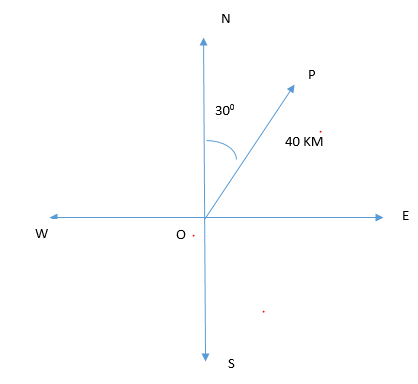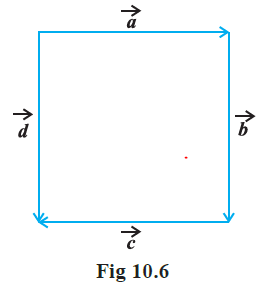# Vector Algebra Exercise 10.1

In this page we have NCERT Solutions for Class 12 Maths Chapter 10: Vector Algebra for EXERCISE 10.1 . Hope you like them and do not forget to like , social share and comment at the end of the page.

Question 1
Graphically represent a 40 km displacement towards 30 o east of north.
Solution$\overrightarrow{OP}$ represent a 40 km displacement towards 30o east of north.

Question 2
Categorize the following measures as vectors and scalars.
(a) 10 kg
(b) 2 meters north - south
(c) 40o
(d) 40 watt
(e) 10-19 coulomb
(f) 20 m / s2
Solution
(a) In 10 kg, only magnitude is involved. So, it is a scalar quantity.
(b) In 2 meters north - south, both the direction and magnitude are involved. So, it is a vector quantity
(c) In 40o, only magnitude is involved. So, it is a scalar quantity.
(d) In 40 watt, only magnitude is involved. So, it is a scalar quantity.
(e) In 10 -19 coulombs, only magnitude is involved. So, it is a scalar quantity.
(f) In 20 m / s2, it is a vector quantity

Question 3
Categorize the following quantities as vector and scalar.
(a) Time period
(b) distance
(c) force
(d) Velocity
(e) work done
Solution
(a) In time period, only magnitude is involved. So, scalar quantity
(b) In distance, only magnitude is involved. So, it is a scalar quantity.
(c) In force, both the direction and magnitude are involved. So, it is a vector quantity
(d) In velocity, both the direction and magnitude are involved. So, it is a vector quantity
(e) In work done, only magnitude is involved. So, it is a scalar quantity.

Question 4: In the following diagram, recognize the corresponding vectors(a) Coinitial
(b) Equal
(c) Collinear but not equal
Solution 4:
(a) We know that Coinitial vectors are those vectors which have same initial point. So, $\overrightarrow{a} \;and\; \overrightarrow{d}$ vectors are coinitial.
(b) We know that Equal vectors are vectors which have same magnitude and direction. So, $\overrightarrow{b} \;and\; \overrightarrow{d}$ vectors are equal.
(c) We know Collinear but not equal are those vectors which are parallel but has different directions. So, $\overrightarrow{a} \;and\; \overrightarrow{c}$ vectors are collinear but not equal.
Question 5:
Check whether the following statements are true or false.
(a)$\overrightarrow{b} \;and\; \overrightarrow{- b}$ vectors are collinear
(b)The magnitudes of the two collinear are always equal.
(c)Collinear vectors are the two vectors having same magnitude.
Solution
(a) True because the two vectors are parallel .
(b)False because collinear vectors must be parallel.
(c)False.

## Related Topics

Go back to Class 12 Main Page using below links